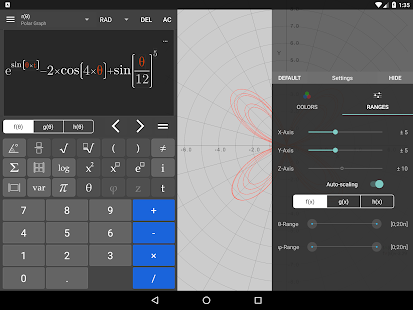Visual Math 4D

Visual Math is a graphical calculator and lets you draw graphs of your mathematical functions in 2D, 3D and 4D with a time-variable (t)

Features :

• draw graphs with one or two variables like f(x), f(x,y)
• visualize your function in 4 dimensions with a time-variable
• compute value tables
• definite integral and derivative – functions
• matrices, vectors, determinante, inverse, transpose,
• trigonometrical functions like sin, cos, tan, sec
• rounding-functions, floor, ceil, round,
• sum and product-function
• save your functions and make screenshots
• modify your graph-range and set customized color options
• rotate and zoom your graph in three dimensions
• display multiple graphs

The app is easy to use and helps students and engineers to visualize their mathematical functions and it can be used as a professional graphical calculator.

What’s New
time-animation, product-function, sum-function, integral, new UI

Screenshots

•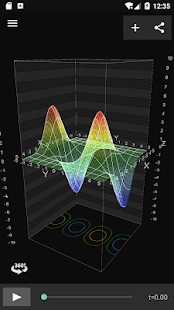•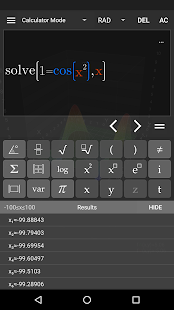•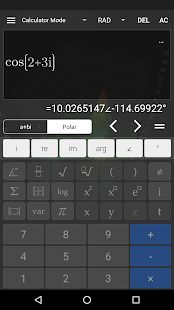•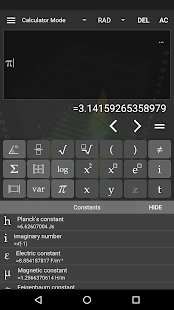•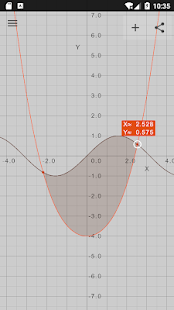•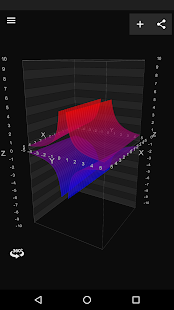•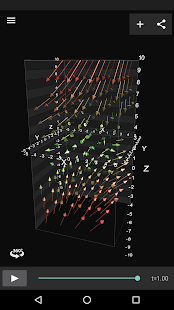•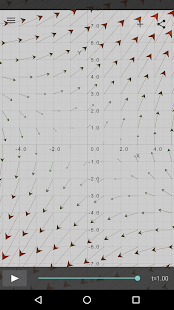•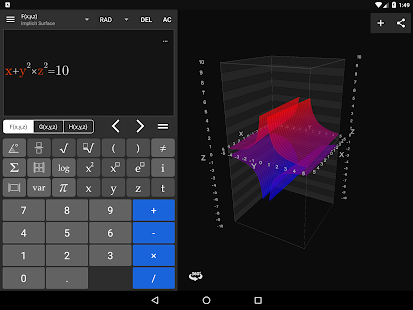•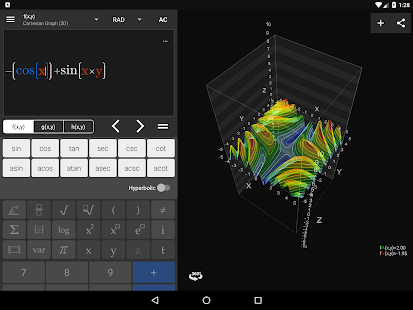•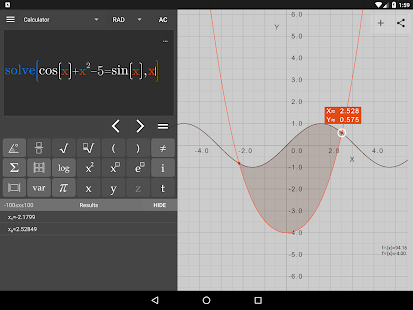•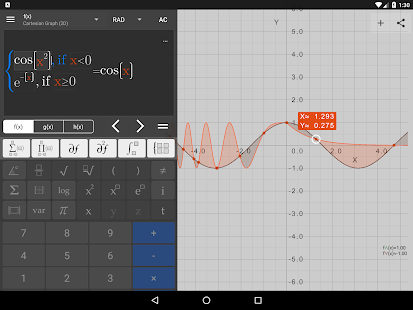•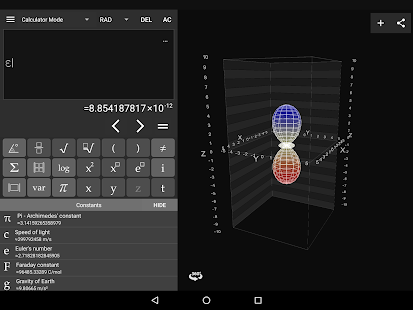•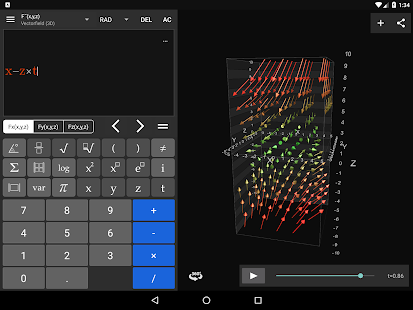•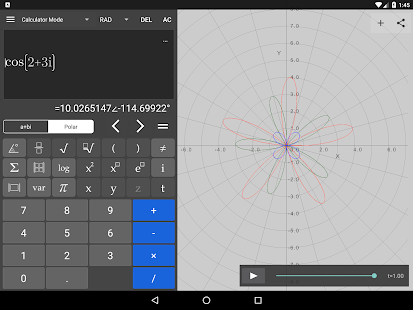•